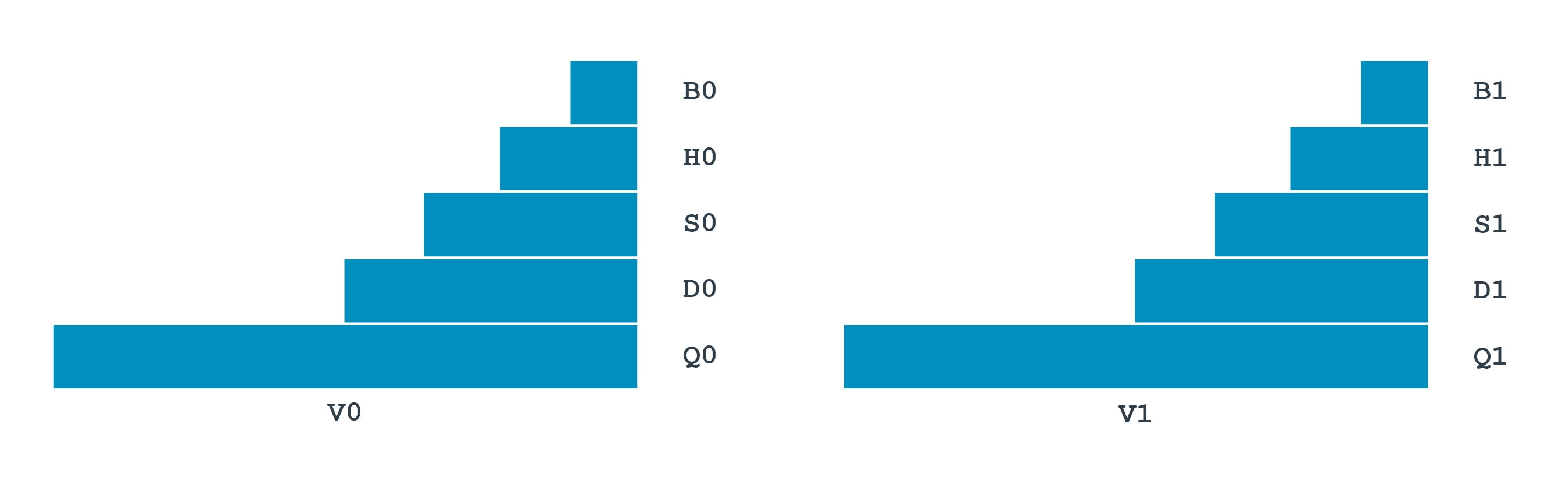## General-purpose registers

Most A64 instructions operate on registers. The architecture provides 31 general purpose registers. Each register can be used as a 64-bit `X` register (`X0..X30`), or as a 32-bit `W` register (`W0..W30`). These are two separate ways of looking at the same register. For example, this register diagram shows that `W0` is the bottom 32 bits of `X0,` and `W1` is the bottom 32 bits of `X1`:For data processing instructions, the choice of `X` or `W` determines the size of the operation. Using `X` registers will result in 64-bit calculations, and using `W` registers will result in 32-bit calculations. This example performs a 32-bit integer addition:

`ADD W0, W1, W2`

This example performs a 64-bit integer addition:

`ADD X0, X1, X2`

When a `W` register is written, as seen in the example above, the top 32 bits of the 64-bit register are zeroed.

There is a separate set of 32 registers used for floating point and vector operations. These registers are 128-bit, but like the general-purpose registers, can be accessed in several ways. `Bx` is 8 bits, `Hx` is 16 bits and so on to `Qx` which is 128 bits.The name you use for the register determines the size of the calculation. This example performs a 32-bit floating point addition:

`FADD S0, S1, S2`

This example performs a 64-bit floating point addition:

`FADD D0, D1, D2`

These registers can also be referred to as `V` registers. When the `V` form is used, the register is treated as being a vector. This means that it is treated as though it contains multiple independent values, instead of a single value. This example performs vector floating point addition:

`FADD V0.2D, V1.2D, V2.2D`

This example performs vector integer addition:

`ADD  V0.2D, V1.2D, V2.2D`

We will look at vector instructions in more detail later in this guide.